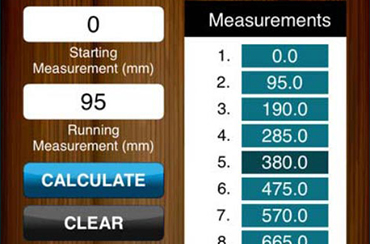This app is highly used by the carpenter, handyman, and material estimator. It contains formula to calculate house square-feet, concrete quantity, stairs, rafter length, spacing, set out spacing and running measurement. More than 30k+ users use app currently.

## Functionality

• Square-feet of house calculation
• Running measurement calculation
• Rafter length calculation
• Spacing and set out spacing calculation
• Concrete quantities calculation
• Stairs needed calculation

## Technology

 Platform iOS Programming Language Swift Database SQLite Tools XCode